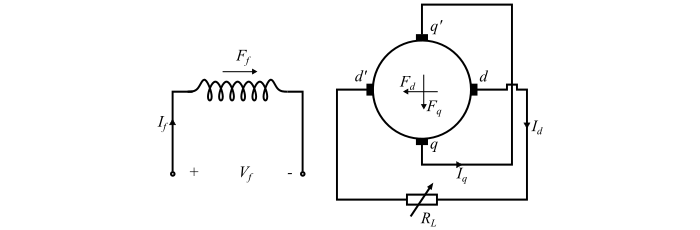# What is a Metadyne? ŌĆō Construction, Working, and Applications

Metadyne is a cross-field machine. The cross-field machines are special DC machines having an additional set of brushes on the direct-axis or d-axis. This arrangement of brushes enables the use of armature MMF to provide most of the excitation and achieve high power gains.

## Construction and Working of Metadyne

An ordinary DC generator can be converted into a metadyne by providing an additional pair of brushes on the direct-axis or d-axis (see the figure). The brushes lie on the quadrature axis or q-axis are short-circuited and the output of the machine is obtained from the d-axis brushes. The stator consists of a control field winding. A field current $­ØÉ╝_{­Øæō}$ flows through the control field winding.When the rotor of the machine is rotating at a constant speed, the MMF of the control field winding (­ØÉ╣­Øæō) induces an EMF ­ØÉĖ­Øæ× between the q-axis brushes qq’. This induced EMF is given by,

$$\mathrm{­ØÉĖ_{­Øæ×} = ­ØÉŠ_{­Øæ×­Øæō}­ØÉ╝_{­Øæō} … (1)}$$

Where, $­ØÉŠ_{­Øæ×­Øæō}$ is a constant.

As the brushes qq’ are short circuited, a q-axis armature current (­ØÉ╝­Øæ×) flows and establishes q-axis MMF (­ØÉ╣­Øæ×). Since the impedance of the short-circuited path is very low, therefore, only a small field current (­ØÉ╝­Øæō) in the control field winding will produce a much larger q-axis armature current. The corresponding flux density wave will be centred on the q-axis. Due to commutator action, this magnetic field is stationary in space. An EMF is induced in the armature by its rotation in the stationary q-axis flux. This generated EMF appears across the daxis brushes dd’ and is given by,

$$\mathrm{­ØÉĖ_{­Øææ} = ­ØÉŠ_{­Øææ­Øæ×}\:­ØÉ╝_{­Øæ×} … (2)}$$

Where, ­ØÉŠ­Øææ­Øæ× is a constant.

Now, if a load of resistance ­Øæģ­ØÉ┐ is connected across the d-axis brushes, the daxis armature current (­ØÉ╝­Øææ) will flow through the load. This current produces d-axis MMF (­ØÉ╣­Øææ). According to the Lenz’s law, the d-axis MMF (­ØÉ╣­Øææ) opposes its cause of production, that is the control field MMF (­ØÉ╣­Øæō).

Each stage of the generation of voltage produces a current whose magnetic field is 90° ahead of the magnetic flux wave producing the voltage. As there are two stages of the voltage generation, thus the MMF of the d-axis output current is shifted by 90° two times, i.e., 180° and hence opposes the control field MMF (­ØÉ╣­Øæō). Therefore, the q-axis generated EMF becomes,

$$\mathrm{­ØÉĖ_{­Øæ×} = ­ØÉŠ_{­Øæ×­Øæō}\:­ØÉ╝_{­Øæō} − ­ØÉŠ_{­Øæ×­Øææ}­ØÉ╝_{­Øææ} … (3)}$$

If the magnetic saturation is neglected and the speed of the machine is assumed to be constant, then ­ØÉŠ­Øæ×­Øææ is a constant.

For a given control field MMF (­ØÉ╣­Øæō) and load resistance, the steady state values of ­ØÉ╝­Øææ and ­ØÉ╝­Øæ× are reached.

From Eqn. (3), it can be seen that any increase in current ­ØÉ╝­Øææ decreases the value of EMF ­ØÉĖ­Øææ. This in turn reduces the current ­ØÉ╝­Øææ. Therefore, ­ØÉĖ­Øææ and ­ØÉ╝­Øææ are decreased. Hence for a given value of control field excitation current (­ØÉ╝­Øæō), the d-axis output current (­ØÉ╝­Øææ) remains constant over a wide range of load variation. Thus, the above discussion shows that a metadyne behaves as a constant current generator.